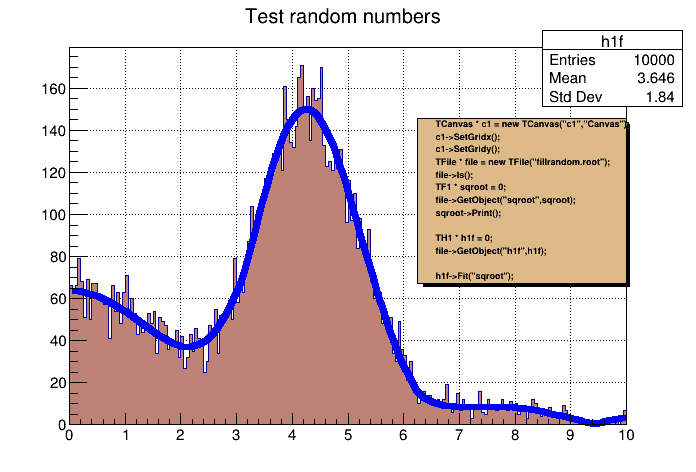# fit1¶

Simple fitting example (1-d histogram with an interpreted function)

Author: Rene Brun
This notebook tutorial was automatically generated with ROOTBOOK-izer from the macro found in the ROOT repository on Saturday, August 13, 2022 at 09:25 AM.

In :
TCanvas *c1 = new TCanvas("c1_fit1","The Fit Canvas",200,10,700,500);
c1->SetGridx();
c1->SetGridy();
c1->GetFrame()->SetFillColor(21);
c1->GetFrame()->SetBorderMode(-1);
c1->GetFrame()->SetBorderSize(5);

gBenchmark->Start("fit1");


We connect the ROOT file generated in a previous tutorial (see Filling histograms with random numbers from a function)

In :
TString dir = gROOT->GetTutorialDir();
dir.Append("/fit/");
TFile *file = nullptr;
if (!gSystem->AccessPathName("fillrandom.root")) {
// file exists
file = TFile::Open("fillrandom.root");
} else {
gROOT->ProcessLine(Form(".x %s../hist/fillrandom.C(0)",dir.Data()));
file = TFile::Open("fillrandom.root");
if (!file) return;
}

Warning in <TFile::Init>: file fillrandom.root probably not closed, trying to recover
Info in <TFile::Recover>: fillrandom.root, recovered key TFormula:form1 at address 226
Info in <TFile::Recover>: fillrandom.root, recovered key TF1:sqroot at address 373
Info in <TFile::Recover>: fillrandom.root, recovered key TH1F:h1f at address 699
Warning in <TFile::Init>: successfully recovered 3 keys


The function "ls()" lists the directory contents of this file

In :
file->ls();

TFile**		fillrandom.root
TFile*		fillrandom.root
KEY: TFormula	form1;1	abs(sin(x)/x)
KEY: TF1	sqroot;1	x*gaus(0) + *form1
KEY: TH1F	h1f;1	Test random numbers


Get object "sqroot" from the file. Undefined objects are searched for using gROOT->FindObject("xxx"), e.g.: TF1 sqroot = (TF1) gROOT.FindObject("sqroot")

In :
TF1 * sqroot = 0;
file->GetObject("sqroot",sqroot);
if (!sqroot){
Error("fit1.C","Cannot find object sqroot of type TF1\n");
return;
}
sqroot->Print();

Formula based function:     sqroot
sqroot : x*gaus(0) + *form1 Ndim= 1, Npar= 4, Number= 0
Formula expression:
x*[p0]*exp(-0.5*((x-[p1])/[p2])*((x-[p1])/[p2]))+[p3]*(abs(sin(x)/x))


Now get and fit histogram h1f with the function sqroot

In :
TH1F* h1f = 0;
file->GetObject("h1f",h1f);
if (!h1f){
Error("fit1.C","Cannot find object h1f of type TH1F\n");
return;
}
h1f->SetFillColor(45);
h1f->Fit("sqroot");

 FCN=198.935 FROM MIGRAD    STATUS=CONVERGED     148 CALLS         149 TOTAL
EDM=2.98567e-07    STRATEGY= 1      ERROR MATRIX ACCURATE
EXT PARAMETER                                   STEP         FIRST
NO.   NAME      VALUE            ERROR          SIZE      DERIVATIVE
1  p0           3.31658e+01   5.45703e-01   3.00376e-03  -1.11540e-03
2  p1           4.00667e+00   1.65304e-02   9.48491e-05  -3.06425e-02
3  p2           9.84663e-01   1.28238e-02   6.05976e-05  -3.04244e-02
4  p3           6.34464e+01   1.33233e+00   8.77483e-03  -3.96109e-04


We now annotate the picture by creating a PaveText object and displaying the list of commands in this macro

In :
TPaveText * fitlabel = new TPaveText(0.6,0.4,0.9,0.75,"NDC");
fitlabel->SetTextAlign(12);
fitlabel->SetFillColor(42);

fit1      : Real Time =   5.01 seconds Cpu Time =   1.83 seconds

gROOT->GetListOfCanvases()->Draw()